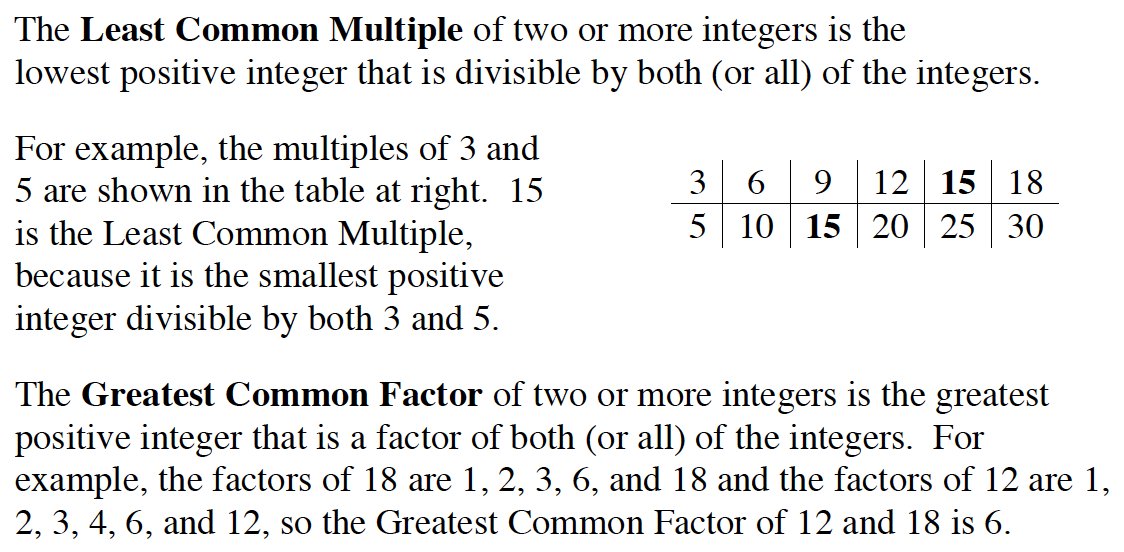Home > CC1MN > Chapter cc21 > Lesson cc21.2.5 > Problem1-104

1-104.

The first four multiples of $5$ are $5$, $10$, $15$, and $20$.   Homework Help ✎

1. What are the first six multiples of $10$?

To find the first six multiples of $10$, you simply multiply 10 by the numbers 1 through 6 separately and create a list of the products you find similar to the list written above.

The first six multiples of 10 are 10, 20, 30, 40, 50, and 60.

2. What are the first six multiples of $8$?

The first six multiples of 8 are 8, 16, 24, 32, 40, and 48.

3. What is the least common multiple of $10$ and $8$?

Refer to the Math Notes box below. It may help to make a table similar to the one in the Math Notes box in order to compare the multiples of 10 and 8.4. What is the greatest common factor of $10$ and $8$?

Refer to the Math Notes box in the hint for part (c). Notice that you will need to find the factors (pairs of numbers that multiply to produce a number) of 10 and 8 to answer this question.

The factors of 10 are: 1, 2, 5, and 10. The factors of 8 are: 1, 2, 4, and 8. Can you find the greatest common factor now?